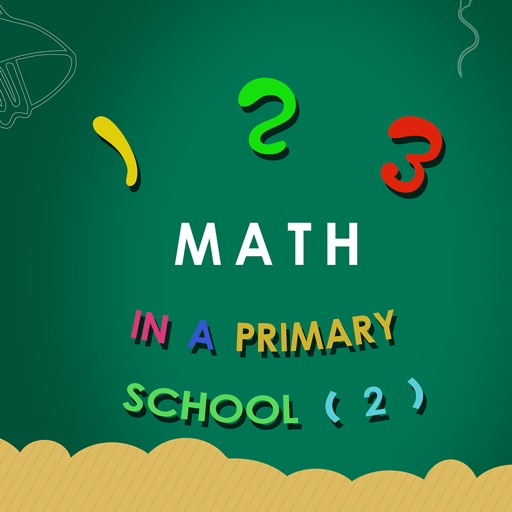## A Complete K-12 math curriculum, from counting to calculus# 123 math in a primary school 2

by mao ye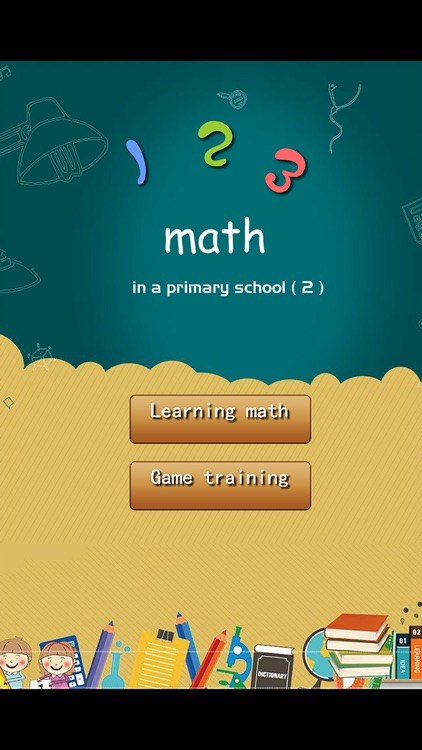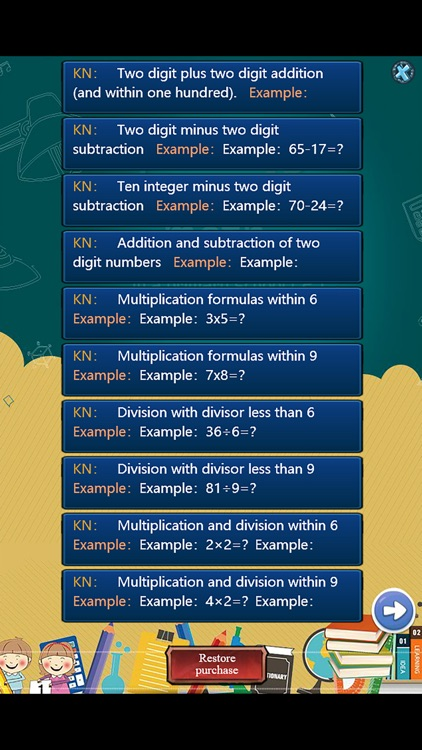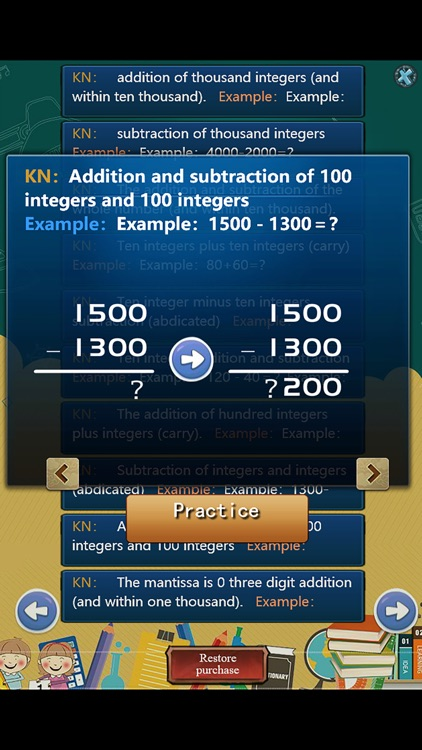A Complete K-12 math curriculum, from counting to calculus.takes the child through addition, subtraction, multiplication, division, and so upward until calculus,This is the second part.### App Details

Version
1.0
Rating
NA
Size
30Mb
Genre
Education Word Trivia
Last updated
August 15, 2018
Release date
August 15, 2018

### App Screenshots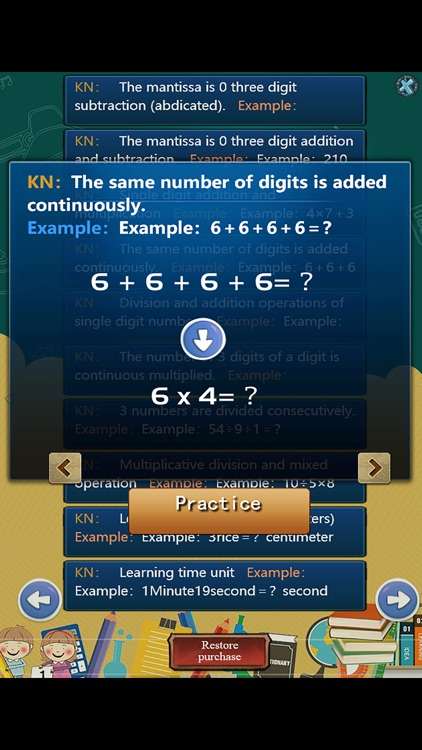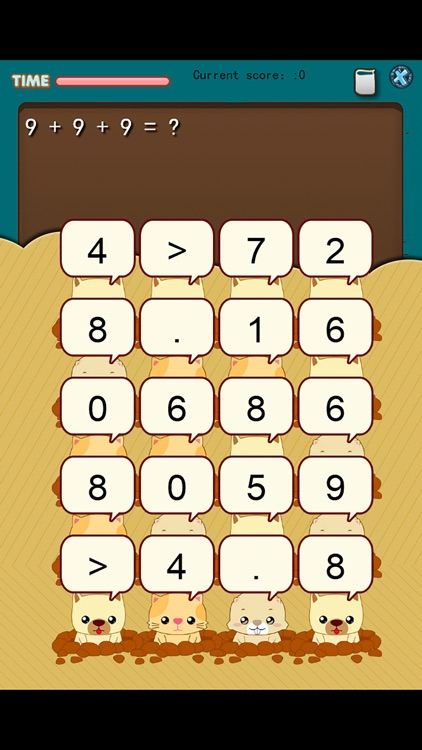### App Store Description

A Complete K-12 math curriculum, from counting to calculus.takes the child through addition, subtraction, multiplication, division, and so upward until calculus,This is the second part.
As you can see, this set of Arithmetic APP is organized in an orderly manner around the discipline of arithmetic itself.

This application includes:

Two digit plus two digit addition (and within one hundred).

Two digit minus two digit subtraction

Ten integer minus two digit subtraction

Addition and subtraction of two digit numbers

Multiplication formulas within 6

Multiplication formulas within 9

Division with divisor less than 6

Division with divisor less than 9

Multiplication and division within 6

Multiplication and division within 9

addition of thousand integers (and within ten thousand).

subtraction of thousand integers

The addition and subtraction of the whole number (and within ten thousand).

Ten integers plus ten integers (carry)

Ten integer minus ten integers subtraction (abdicated)

The addition of hundred integers plus integers (carry).

Subtraction of integers and integers (abdicated)

Addition and subtraction of 100 integers and 100 integers

The mantissa is 0 three digit addition (and within one thousand).

The mantissa is 0 three digit subtraction (abdicated).

The mantissa is 0 three digit addition and subtraction.

The same number of digits is added continuously.

Division and addition operations of single digit numbers

The number of 3 digits of a digit is continuous multiplied.

3 numbers are divided consecutively.

Multiplicative division and mixed operation

Length units (meters, centimeters)

Learning time unit

Comparing the length of time

Division of remainder

Numeric calculation with parentheses

Disclaimer:
AppAdvice does not own this application and only provides images and links contained in the iTunes Search API, to help our users find the best apps to download. If you are the developer of this app and would like your information removed, please send a request to [email protected] and your information will be removed.